# Pen and pencil

Cost of a pen is Rs. 12 3/5 and cost of a pencil is Rs. 3 2/5. What is the total cost of pen and pencil?

x =  16 Rs

### Step-by-step explanation: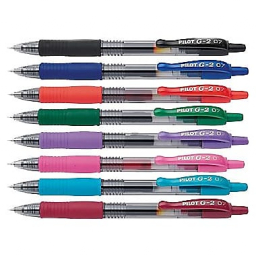Did you find an error or inaccuracy? Feel free to write us. Thank you!## Related math problems and questions:3 3/4 + 2 3/5 + 5 1/2 Show your solution.
• The sum 12The sum of 3 mixed numbers is 20 13/15. two of the numbers are 6 1/3 and 7 5/6. what is the third number?
• Fractions and mixed numerals(a) Convert the following mixed numbers to improper fractions. i. 3 5/8 ii. 7 7/6 (b) Convert the following improper fraction to mixed number. i. 13/4 ii. 78/5 (c) Simplify these fractions to their lowest terms. i. 36/42 ii. 27/45 2. evaluate following ex
• Packets weight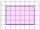The weight of three packets are 3 2/5 kg + 2 1/3 kg + 5 1/2 kg. Find total weight of all the three packets.
• The cost 3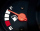The cost of 2 ¾ litres of petrol is Rs 250 ½. What is the cost of 1 litre of petrol?
• Painting classroomsMartha solicited 3 2/3 liters of paint for the brigade. Their city mayor gave their school another 2 2/5 liters of paint. If each classroom needs 1 3/7 liters, how many classrooms can be painted?
• Product increased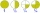When the product of 2/3 and 6/10 is increased by 2/5, the result is?
• Three rectangles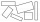Some wire is used to make 3 rectangles: A, B, and C. Rectangle B's dimensions are 3/5 cm larger than Rectangle A's dimensions, and Rectangle C's dimensions are again 3/5 cm larger than Rectangle B's dimensions. Rectangle A is 2 cm by 3 1/5 cm. What is the
• A shopkeeper 3A shopkeeper sells 8 1/3 kg, 10 1/4 kg and 11 1/5 kg of apples on 3 consecutive days. What is the total weight of apples sold?
• Ingrid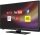Ingrid watched television for 2 1/4 hours, and Devon watched for 3 2/3 hours. For how many hours did they watch television in total?Add two mixed fractions: 2 4/6 + 1 3/65 2/5 hours a week  mathematics,  3 3/4 hours a week   Natural sciences, 4 3/8 hours a week  Technology . how many hours does  he spend on social sciences if he spend 17 1/2 hours a week for the four subject?A farmer's truck is carrying 4 2/3 tons of hay and straw. If 3 2/5 tons of the load is hay, how much straw is the truck carrying?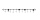What is the rational number between 2 1/4 and 2 4/5?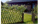A certain type of fencing sells for \$9 a yard. How much will 10 2/3 yards of fencing cost?The difference  of 5 1/2 and 2/3 is added to the product of 5/6 and 1/2Add this two mixed numbers: 1 5/6 + 2 2/11=